Next: Examples of Jensen inequalities Up: Entropy and Jensen inequality Previous: Entropy and Jensen inequality

# THE JENSEN INEQUALITY

Let f be a function with a positive second derivative. Such a function is called convex" and satisfies the inequality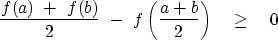(1)
Equation (1) relates a function of an average to an average of the function. The average can be weighted, for example,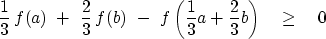(2)
Figure 1 is a graphical interpretation of equation (2) for the function f=x2.

 jen Figure 1 Sketch of y=x2 for interpreting equation ((2)).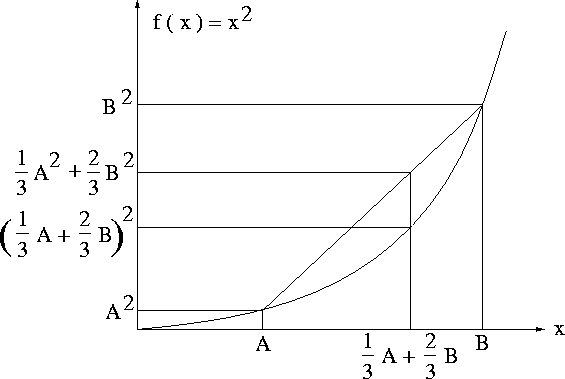There is nothing special about f=x2, except that it is convex. Given three numbers a, b, and c, the inequality (2) can first be applied to a and b, and then to c and the average of a and b. Thus, recursively, an inequality like (2) can be built for a weighted average of three or more numbers. Define weights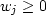that are normalized (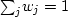). The general result is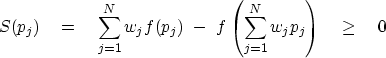(3)
If all the pj are the same, then both of the two terms in S are the same, and S vanishes. Hence, minimizing S is like urging all the pj to be identical. Equilibrium is when S is reduced to the smallest possible value which satisfies any constraints that may be applicable. The function S defined by (3) is like the entropy defined in thermodynamics.Next: Examples of Jensen inequalities Up: Entropy and Jensen inequality Previous: Entropy and Jensen inequality
Stanford Exploration Project
10/21/1998